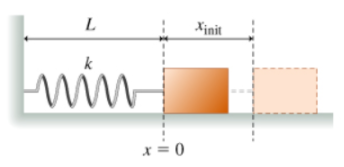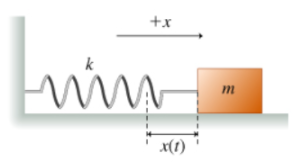# Problem: Learning Goal: To understand the application of the general harmonic equation to finding the acceleration of a spring oscillator as a function of time.One end of a spring with spring constant k is attached to the wall. The other end is attached to a block of mass m. The block rests on a frictionless horizontal surface. The equilibrium position of the left side of the block is defined to be x = 0. The length of the relaxed spring is L.(Figure 1)The block is slowly pulled from its equilibrium position to some position xinit &gt; 0 along the x axis. At time t = 0, the block is released with zero initial velocity.The goal of this problem is to determine the acceleration of the block a(t) as a function of time in terms of k, m, and xinit.It is known that a general solution for the position of a harmonic oscillator isx(t) = C cos(ωt) + S sin(ωt),where C, S, and ω are constants. (Figure 2)Your task, therefore, is to determine the values of C, S, and ω in terms of k, m, and xinit and then use the connection between x(t) and a(t) to find the acceleration.QUESTION: Using the fact that acceleration is the second derivative of position, find the acceleration of the block a(t) as a function of time. Express your answer in terms of ω, t, and x(t).

###### FREE Expert Solution

Derivative of sine/cosine:

$\overline{)\begin{array}{rcl}\frac{\mathbf{d}}{\mathbf{d}\mathbf{t}}\mathbf{s}\mathbf{i}\mathbf{n}\mathbf{\left(}\mathbf{a}\mathbf{t}\mathbf{\right)}& {\mathbf{=}}& \mathbf{a}\mathbf{c}\mathbf{o}\mathbf{s}\mathbf{\left(}\mathbf{a}\mathbf{t}\mathbf{\right)}\\ \frac{\mathbf{d}}{\mathbf{d}\mathbf{t}}\mathbf{c}\mathbf{o}\mathbf{s}\mathbf{\left(}\mathbf{a}\mathbf{t}\mathbf{\right)}& {\mathbf{=}}& \mathbf{-}\mathbf{a}\mathbf{s}\mathbf{i}\mathbf{n}\mathbf{\left(}\mathbf{a}\mathbf{t}\mathbf{\right)}\end{array}}$

We'll determine the derivatives then substitute initial conditions to solve for C and S.

x(t) = C cos(ωt) + S sin(ωt)

v(t) = (d/dt)x(t) = (d/dt)(C cos(ωt) + S sin(ωt)) = -ωC sin(ωt) + ωS cos(ωt)

85% (220 ratings)###### Problem Details

Learning Goal: To understand the application of the general harmonic equation to finding the acceleration of a spring oscillator as a function of time.

One end of a spring with spring constant k is attached to the wall. The other end is attached to a block of mass m. The block rests on a frictionless horizontal surface. The equilibrium position of the left side of the block is defined to be x = 0. The length of the relaxed spring is L.(Figure 1)The block is slowly pulled from its equilibrium position to some position xinit > 0 along the x axis. At time t = 0, the block is released with zero initial velocity.

The goal of this problem is to determine the acceleration of the block a(t) as a function of time in terms of k, m, and xinit.

It is known that a general solution for the position of a harmonic oscillator is

x(t) = C cos(ωt) + S sin(ωt),

where C, S, and ω are constants. (Figure 2)Your task, therefore, is to determine the values of C, S, and ω in terms of k, m, and xinit and then use the connection between x(t) and a(t) to find the acceleration.

QUESTION: Using the fact that acceleration is the second derivative of position, find the acceleration of the block a(t) as a function of time. Express your answer in terms of ω, t, and x(t).